## An easy to use calculator with steps that solves quadratic equations in the form ax^2 + bx + c = 0, ax^2 + bx = 0 and ax^2 + c = 0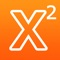# Quadratic Equation Calculator with Steps

by Intemodino Group s.r.o.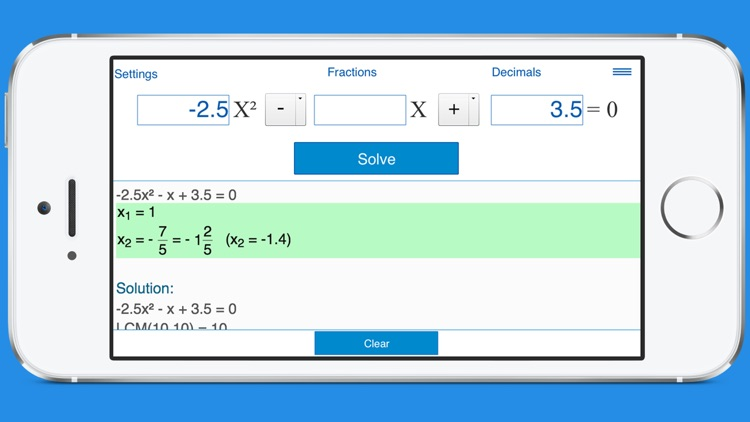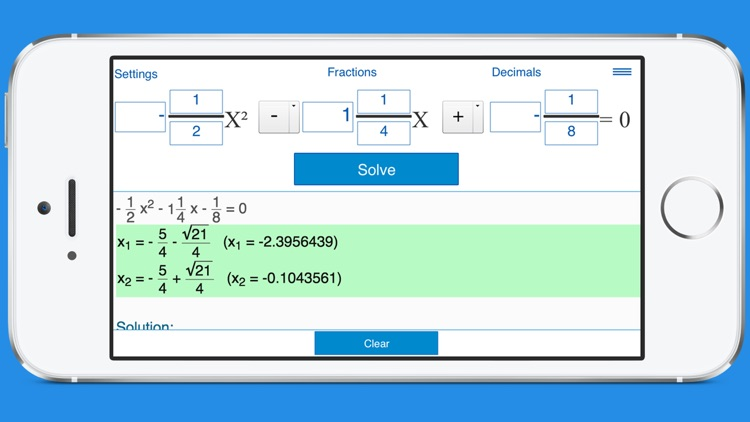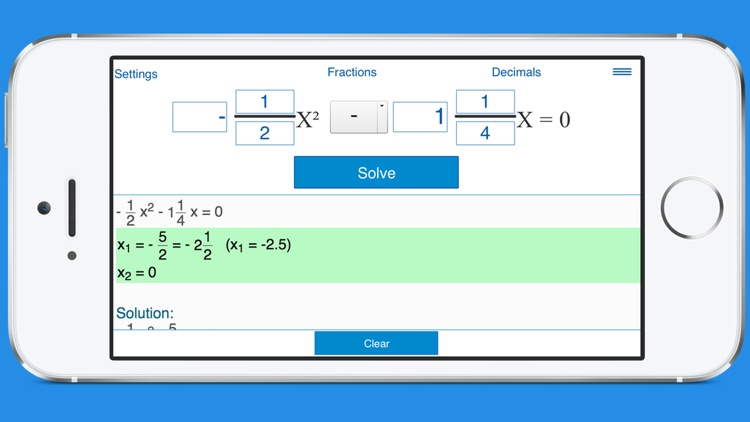An easy to use calculator with steps that solves quadratic equations in the form ax^2 + bx + c = 0, ax^2 + bx = 0 and ax^2 + c = 0. Just enter the values of ‘a’, ‘b’ and ‘c’, and click ‘Solve’.### App Details

Version
2.0.3
Rating
NA
Size
22Mb
Genre
Education Utilities
Last updated
March 2, 2016
Release date
September 11, 2012

### App Screenshots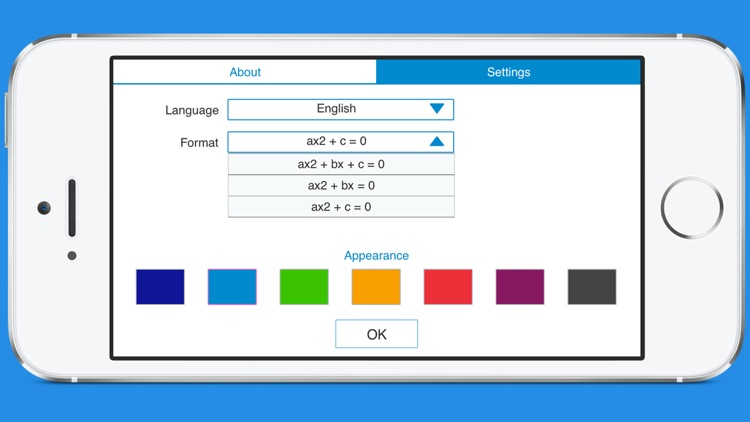### App Store Description

An easy to use calculator with steps that solves quadratic equations in the form ax^2 + bx + c = 0, ax^2 + bx = 0 and ax^2 + c = 0. Just enter the values of ‘a’, ‘b’ and ‘c’, and click ‘Solve’.

Features:

• Solves quadratic equations with real and complex roots using the quadratic formula.

• Shows steps.

• Supports whole, fractional and decimal coefficients.

• Supports pre-defined formats for complete and incomplete quadratic equations.

• History tape to view recent calculations.

Disclaimer:
AppAdvice does not own this application and only provides images and links contained in the iTunes Search API, to help our users find the best apps to download. If you are the developer of this app and would like your information removed, please send a request to [email protected] and your information will be removed.